The MCAT CARS Strategy Course begins February 27!

### Periodic Motion

Written by Ryan on Jan 5, 2020

## Period and Frequency

The period is the duration of one cycle in a repeating event, while the frequency is the number of cycles per unit time.

### LEARNING OBJECTIVES

Practice converting between frequency and period

### KEY TAKEAWAYS

#### Key Points

• Motion that repeats itself regularly is called periodic motion. One complete repetition of the motion is called a cycle. The duration of each cycle is the period.
• The frequency refers to the number of cycles completed in an interval of time. It is the reciprocal of the period and can be calculated with the equation f=1/T.
• Some motion is best characterized by the angular frequency (ω). The angular frequency refers to the angular displacement per unit time and is calculated from the frequency with the equation ω=2πf.

#### Key Terms

• period: The duration of one cycle in a repeating event.
• angular frequency: The angular displacement per unit time.
• frequency: The quotient of the number of times n a periodic phenomenon occurs over the time t in which it occurs: f = n / t.

### Period and Frequency

The usual physics terminology for motion that repeats itself over and over is periodic motion, and the time required for one repetition is called the period, often expressed as the letter T. (The symbol P is not used because of the possible confusion with momentum. ) One complete repetition of the motion is called a cycle. The frequency is defined as the number of cycles per unit time. Frequency is usually denoted by a Latin letter f or by a Greek letter ν (nu). Note that period and frequency are reciprocals of each other.Sinusoidal Waves of Varying Frequencies: Sinusoidal waves of various frequencies; the bottom waves have higher frequencies than those above. The horizontal axis represents time.

$\text{f}=1/\text{T}$

For example, if a newborn baby’s heart beats at a frequency of 120 times a minute, its period (the interval between beats) is half a second. If you calibrate your intuition so that you expect large frequencies to be paired with short periods, and vice versa, you may avoid some embarrassing mistakes on physics exams.

### UnitsLocomotive Wheels: The locomotive’s wheels spin at a frequency of f cycles per second, which can also be described as ω radians per second. The mechanical linkages allow the linear vibration of the steam engine’s pistons, at frequency f, to drive the wheels.

In SI units, the unit of frequency is the hertz (Hz), named after the German physicist Heinrich Hertz: 1 Hz indicates that an event repeats once per second. A traditional unit of measure used with rotating mechanical devices is revolutions per minute, abbreviated RPM. 60 RPM equals one hertz (i.e., one revolution per second, or a period of one second). The SI unit for period is the second.

### Angular Frequency

Often periodic motion is best expressed in terms of angular frequency, represented by the Greek letter ω (omega). Angular frequency refers to the angular displacement per unit time (e.g., in rotation) or the rate of change of the phase of a sinusoidal waveform (e.g., in oscillations and waves), or as the rate of change of the argument of the sine function.

$\text{y}\left(\text{t}\right)=\text{sin}\left(\theta \left(\text{t}\right)\right)=\text{sin}\left(\omega \text{t}\right)=\text{sin}\left(2\pi \text{ft}\right)$

$\omega =2\pi \text{f}$

Angular frequency is often represented in units of radians per second (recall there are 2π radians in a circle).

## Period of a Mass on a Spring

The period of a mass m on a spring of spring constant k can be calculated as

$\text{T}=2\pi \sqrt{\frac{\text{m}}{\text{k}}}$

.

### LEARNING OBJECTIVES

Identify parameters necessary to calculate the period and frequency of an oscillating mass on the end of an ideal spring

### KEY TAKEAWAYS

#### Key Points

• If an object is vibrating to the right and left, then it must have a leftward force on it when it is on the right side, and a rightward force when it is on the left side.
• The restoring force causes an oscillating object to move back toward its stable equilibrium position, where the net force on it is zero.
• The simplest oscillations occur when the restoring force is directly proportional to displacement. In this case the force can be calculated as F=-kx, where F is the restoring force, k is the force constant, and x is the displacement.
• The motion of a mass on a spring can be described as Simple Harmonic Motion (SHM): oscillatory motion that follows Hooke’s Law.
• The period of a mass on a spring is given by the equation
$\text{T}=2\pi \sqrt{\frac{\text{m}}{\text{k}}}$

#### Key Terms

• Restoring force: A variable force that gives rise to an equilibrium in a physical system. If the system is perturbed away from the equilibrium, the restoring force will tend to bring the system back toward equilibrium. The restoring force is a function only of position of the mass or particle. It is always directed back toward the equilibrium position of the system
• amplitude: The maximum absolute value of some quantity that varies.

### Understanding the Restoring Force

Newton’s first law implies that an object oscillating back and forth is experiencing forces. Without force, the object would move in a straight line at a constant speed rather than oscillate. It is important to understand how the force on the object depends on the object’s position. If an object is vibrating to the right and left, then it must have a leftward force on it when it is on the right side, and a rightward force when it is on the left side. In one dimension, we can represent the direction of the force using a positive or negative sign, and since the force changes from positive to negative there must be a point in the middle where the force is zero. This is the equilibrium point, where the object would stay at rest if it was released at rest. It is common convention to define the origin of our coordinate system so that x equals zero at equilibrium.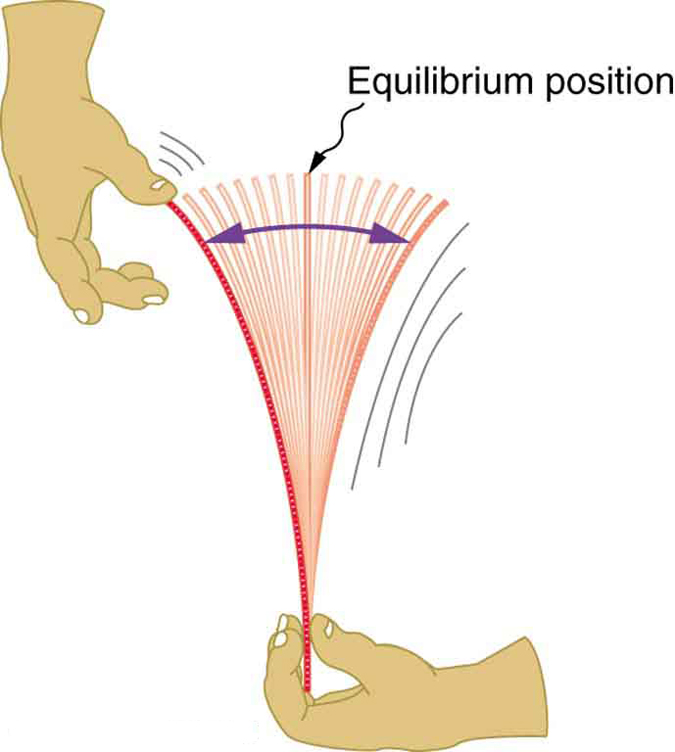Oscillating Ruler: When displaced from its vertical equilibrium position, this plastic ruler oscillates back and forth because of the restoring force opposing displacement. When the ruler is on the left, there is a force to the right, and vice versa.

Consider, for example, plucking a plastic ruler shown in the first figure. The deformation of the ruler creates a force in the opposite direction, known as a restoring force. Once released, the restoring force causes the ruler to move back toward its stable equilibrium position, where the net force on it is zero. However, by the time the ruler gets there, it gains momentum and continues to move to the right, producing the opposite deformation. It is then forced to the left, back through equilibrium, and the process is repeated until dissipative forces (e.g., friction) dampen the motion. These forces remove mechanical energy from the system, gradually reducing the motion until the ruler comes to rest.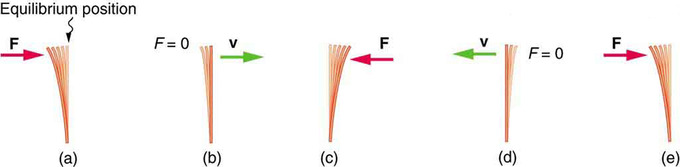Restoring force, momentum, and equilibrium: (a) The plastic ruler has been released, and the restoring force is returning the ruler to its equilibrium position. (b) The net force is zero at the equilibrium position, but the ruler has momentum and continues to move to the right. (c) The restoring force is in the opposite direction. It stops the ruler and moves it back toward equilibrium again. (d) Now the ruler has momentum to the left. (e) In the absence of damping (caused by frictional forces), the ruler reaches its original position. From there, the motion will repeat itself.

### Hooke’s Law

The simplest oscillations occur when the restoring force is directly proportional to displacement. The name that was given to this relationship between force and displacement is Hooke’s law:

$\text{F}=\text{kx}$

Here, F is the restoring force, x is the displacement from equilibrium or deformation, and k is a constant related to the difficulty in deforming the system (often called the spring constant or force constant). Remember that the minus sign indicates the restoring force is in the direction opposite to the displacement. The force constant k is related to the rigidity (or stiffness) of a system—the larger the force constant, the greater the restoring force, and the stiffer the system. The units of k are newtons per meter (N/m). For example, k is directly related to Young’s modulus when we stretch a string. A typical physics laboratory exercise is to measure restoring forces created by springs, determine if they follow Hooke’s law, and calculate their force constants if they do.

Mass on a Spring

A common example of an objecting oscillating back and forth according to a restoring force directly proportional to the displacement from equilibrium (i.e., following Hooke’s Law) is the case of a mass on the end of an ideal spring, where “ideal” means that no messy real-world variables interfere with the imagined outcome.

The motion of a mass on a spring can be described as Simple Harmonic Motion (SHM), the name given to oscillatory motion for a system where the net force can be described by Hooke’s law. We can now determine how to calculate the period and frequency of an oscillating mass on the end of an ideal spring. The period T can be calculated knowing only the mass, m, and the force constant, k:

$\text{T}=2\pi \sqrt{\frac{\text{m}}{\text{k}}}$

When dealing with

$\text{f}=1/\text{T}$

, the frequency is given by:

$\text{f}=\frac{1}{2\pi }\sqrt{\frac{\text{k}}{\text{m}}}$

We can understand the dependence of these equations on m and k intuitively. If one were to increase the mass on an oscillating spring system with a given k, the increased mass will provide more inertia, causing the acceleration due to the restoring force F to decrease (recall Newton’s Second Law:

$\text{F}=\text{ma}$

). This will lengthen the oscillation period and decrease the frequency. In contrast, increasing the force constant k will increase the restoring force according to Hooke’s Law, in turn causing the acceleration at each displacement point to also increase. This reduces the period and increases the frequency. The maximum displacement from equilibrium is known as the amplitude X.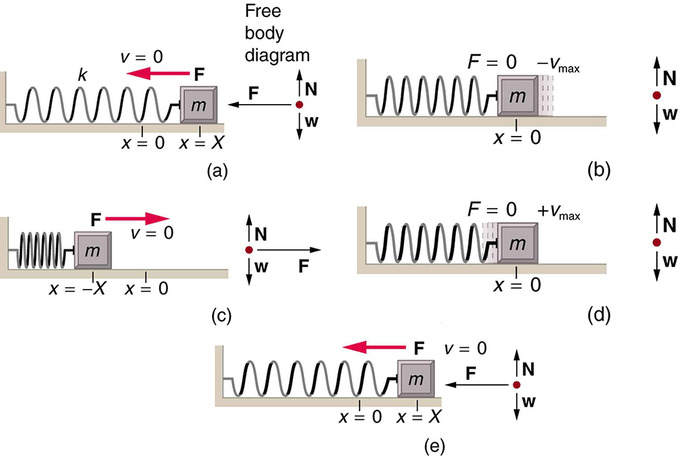Motion of a mass on an ideal spring: An object attached to a spring sliding on a frictionless surface is an uncomplicated simple harmonic oscillator. When displaced from equilibrium, the object performs simple harmonic motion that has an amplitude X and a period T. The object’s maximum speed occurs as it passes through equilibrium. The stiffer the spring is, the smaller the period T. The greater the mass of the object is, the greater the period T. (a) The mass has achieved its greatest displacement X to the right and now the restoring force to the left is at its maximum magnitude. (b) The restoring force has moved the mass back to its equilibrium point and is now equal to zero, but the leftward velocity is at its maximum. (c) The mass’s momentum has carried it to its maximum displacement to the right. The restoring force is now to the right, equal in magnitude and opposite in direction compared to (a). (d) The equilibrium point is reach again, this time with momentum to the right. (e) The cycle repeats.

## Simple Harmonic Motion

Simple harmonic motion is a type of periodic motion where the restoring force is directly proportional to the displacement.

### LEARNING OBJECTIVES

Relate the restoring force and the displacement during the simple harmonic motion

### KEY TAKEAWAYS

#### Key Points

• Simple harmonic motion is often modeled with the example of a mass on a spring, where the restoring force obey’s Hooke’s Law and is directly proportional to the displacement of an object from its equilibrium position.
• Any system that obeys simple harmonic motion is known as a simple harmonic oscillator.
• The equation of motion that describes simple harmonic motion can be obtained by combining Newton’s Second Law and Hooke’s Law into a second-order linear ordinary differential equation:
${\text{F}}_{\text{net}}=\text{m}\frac{{\text{d}}^{2}\text{x}}{{\text{dt}}^{2}}=-\text{kx}$

.

#### Key Terms

• simple harmonic oscillator: A device that implements Hooke’s law, such as a mass that is attached to a spring, with the other end of the spring being connected to a rigid support, such as a wall.
• oscillator: A pattern that returns to its original state, in the same orientation and position, after a finite number of generations.

### Simple Harmonic Motion

Simple harmonic motion is a type of periodic motion where the restoring force is directly proportional to the displacement (i.e., it follows Hooke’s Law). It can serve as a mathematical model of a variety of motions, such as the oscillation of a spring. In addition, other phenomena can be approximated by simple harmonic motion, such as the motion of a simple pendulum, or molecular vibration.

Simple Harmonic Motion: A brief introduction to simple harmonic motion for calculus-based physics students.

Simple harmonic motion is typified by the motion of a mass on a spring when it is subject to the linear elastic restoring force given by Hooke’s Law. A system that follows simple harmonic motion is known as a simple harmonic oscillator.

### Dynamics of Simple Harmonic Oscillation

For one-dimensional simple harmonic motion, the equation of motion (which is a second-order linear ordinary differential equation with constant coefficients) can be obtained by means of Newton’s second law and Hooke’s law.

${\text{F}}_{\text{net}}=\text{m}\frac{{\text{d}}^{2}\text{x}}{{\text{dt}}^{2}}=-\text{kx}$

,

where m is the mass of the oscillating body, x is its displacement from the equilibrium position, and k is the spring constant. Therefore:

$\frac{{\text{d}}^{2}\text{x}}{{\text{dt}}^{2}}=-\left(\frac{\text{k}}{\text{m}}\right)\text{x}$

.

Solving the differential equation above, a solution which is a sinusoidal function is obtained.

$\text{x}\left(\text{t}\right)={\text{c}}_{1}\text{cos}\left(\omega \text{t}\right)+{\text{c}}_{2}\text{sin}\left(\omega \text{t}\right)=\text{Acos}\left(\omega \text{t}-\phi \right)$

,

where

$\omega =\sqrt{\frac{\text{k}}{\text{m}}}$

,

$\text{A}=\sqrt{{\text{c}}_{1}^{2}+{\text{c}}_{2}^{2}}$

,

$tan\phi =\left(\frac{{\text{c}}_{2}}{{\text{c}}_{1}}\right)$

.

In the solution, c1 and c2 are two constants determined by the initial conditions, and the origin is set to be the equilibrium position. Each of these constants carries a physical meaning of the motion: A is the amplitude (maximum displacement from the equilibrium position), ω = 2πf is the angular frequency, and φ is the phase.

We can use differential calculus and find the velocity and acceleration as a function of time:

$\text{v}\left(\text{t}\right)=\frac{\text{dx}}{\text{dt}}=-\text{A}\omega \text{sin}\left(\omega \text{t}-\phi \right)$

$\text{a}\left(\text{t}\right)=\frac{{\text{d}}^{2}\text{x}}{{\text{dt}}^{2}}=-\text{A}{\omega }^{2}\text{cos}\left(\omega \text{t}-\phi \right)$

.

Acceleration can also be expressed as a function of displacement:

$\text{a}\left(\text{t}\right)=-{\omega }^{2}\text{x}$

.

Then since ω = 2πf,

$\text{f}=\frac{1}{2\pi }\sqrt{\frac{\text{k}}{\text{m}}}$

.

Recalling that

$\text{T}=1/\text{f}$

,

$\text{T}=2\pi \sqrt{\frac{\text{m}}{\text{k}}}$

.

Using Newton’s Second Law, Hooke’s Law, and some differential Calculus, we were able to derive the period and frequency of the mass oscillating on a spring that we encountered in the last section! Note that the period and frequency are completely independent of the amplitude.

The below figure shows the simple harmonic motion of an object on a spring and presents graphs of x(t),v(t), and a(t) versus time. You should learn to create mental connections between the above equations, the different positions of the object on a spring in the cartoon, and the associated positions in the graphs of x(t), v(t), and a(t).Visualizing Simple Harmonic Motion: Graphs of x(t),v(t), and a(t) versus t for the motion of an object on a spring. The net force on the object can be described by Hooke’s law, and so the object undergoes simple harmonic motion. Note that the initial position has the vertical displacement at its maximum value X; v is initially zero and then negative as the object moves down; and the initial acceleration is negative, back toward the equilibrium position and becoming zero at that point.

## Simple Harmonic Motion and Uniform Circular Motion

Simple harmonic motion is produced by the projection of uniform circular motion onto one of the axes in the x-y plane.

### LEARNING OBJECTIVES

Describe relationship between the simple harmonic motion and uniform circular motion

### KEY TAKEAWAYS

#### Key Points

• Uniform circular motion describes the movement of an object traveling a circular path with constant speed. The one-dimensional projection of this motion can be described as simple harmonic motion.
• In uniform circular motion, the velocity vector v is always tangent to the circular path and constant in magnitude. The acceleration is constant in magnitude and points to the center of the circular path, perpendicular to the velocity vector at every instant.
• If an object moves with angular velocity ω around a circle of radius r centered at the origin of the x-y plane, then its motion along each coordinate is simple harmonic motion with amplitude r and angular frequency ω.

#### Key Terms

• centripetal acceleration: Acceleration that makes a body follow a curved path: it is always perpendicular to the velocity of a body and directed towards the center of curvature of the path.
• uniform circular motion: Movement around a circular path with constant speed.

### Uniform Circular Motion

Uniform circular motion describes the motion of a body traversing a circular path at constant speed. The distance of the body from the center of the circle remains constant at all times. Though the body’s speed is constant, its velocity is not constant: velocity (a vector quantity) depends on both the body’s speed and its direction of travel. Since the body is constantly changing direction as it travels around the circle, the velocity is changing also. This varying velocity indicates the presence of an acceleration called the centripetal acceleration. Centripetal acceleration is of constant magnitude and directed at all times towards the center of the circle. This acceleration is, in turn, produced by a centripetal force —a force in constant magnitude, and directed towards the center.

### Velocity

The above figure illustrates velocity and acceleration vectors for uniform motion at four different points in the orbit. Since velocity v is tangent to the circular path, no two velocities point in the same direction. Although the object has a constant speed, its direction is always changing. This change in velocity is due to an acceleration, a, whose magnitude is (like that of the velocity) held constant, but whose direction also is always changing. The acceleration points radially inwards (centripetally) and is perpendicular to the velocity. This acceleration is known as centripetal acceleration.Uniform Circular Motion (at Four Different Point in the Orbit): Velocity v and acceleration a in uniform circular motion at angular rate ω; the speed is constant, but the velocity is always tangent to the orbit; the acceleration has constant magnitude, but always points toward the center of rotation

Displacement around a circular path is often given in terms of an angle θ. This angle is the angle between a straight line drawn from the center of the circle to the objects starting position on the edge and a straight line drawn from the objects ending position on the edge to center of the circle. See for a visual representation of the angle where the point p started on the x- axis and moved to its present position. The angle θ describes how far it moved.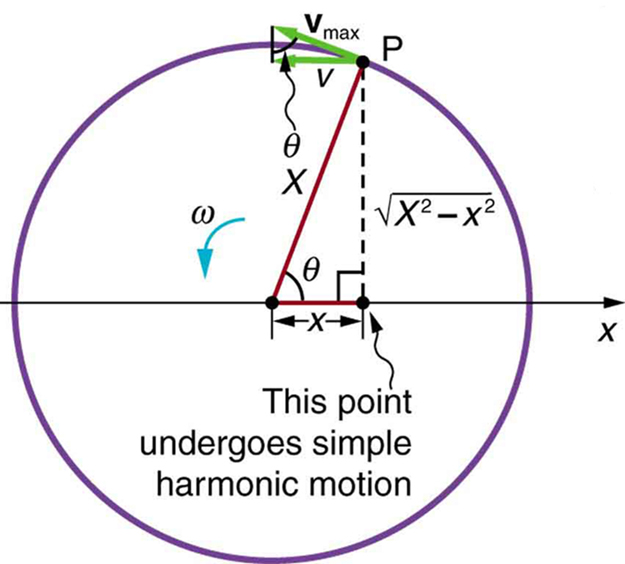Projection of Uniform Circular Motion: A point P moving on a circular path with a constant angular velocity ω is undergoing uniform circular motion. Its projection on the x-axis undergoes simple harmonic motion. Also shown is the velocity of this point around the circle, v−max, and its projection, which is v. Note that these velocities form a similar triangle to the displacement triangle.

For a path around a circle of radius r, when an angle θ (measured in radians ) is swept out, the distance traveled on the edge of the circle is s = rθ. You can prove this yourself by remembering that the circumference of a circle is 2*pi*r, so if the object traveled around the whole circle (one circumference) it will have gone through an angle of 2pi radians and traveled a distance of 2pi*r. Therefore, the speed of travel around the orbit is:

$\text{v}=\text{r}\frac{\text{d}\theta }{\text{dt}}=\text{r}\omega$

,

where the angular rate of rotation is ω. (Note that ω = v/r. ) Thus, v is a constant, and the velocity vector v also rotates with constant magnitude v, at the same angular rate ω.

### Acceleration

The acceleration in uniform circular motion is always directed inward and is given by:

$\text{a}=\text{v}\frac{\text{d}\theta }{\text{dt}}=\text{v}\omega =\frac{{\text{v}}^{2}}{\text{r}}$

.

This acceleration acts to change the direction of v, but not the speed.

### Simple Harmonic Motion from Uniform Circular Motion

There is an easy way to produce simple harmonic motion by using uniform circular motion. The figure below demonstrates one way of using this method. A ball is attached to a uniformly rotating vertical turntable, and its shadow is projected onto the floor as shown. The shadow undergoes simple harmonic motion.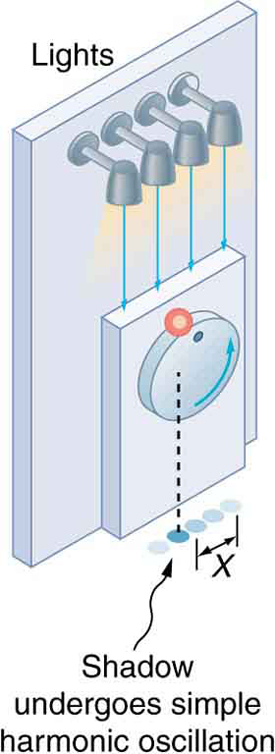Shadow of a Ball Undergoing Simple Harmonic Motion: The shadow of a ball rotating at constant angular velocity ω on a turntable goes back and forth in precise simple harmonic motion.

The next figure shows the basic relationship between uniform circular motion and simple harmonic motion. The point P travels around the circle at constant angular velocity ω. The point P is analogous to the ball on a turntable in the figure above. The projection of the position of P onto a fixed axis undergoes simple harmonic motion and is analogous to the shadow of the object. At a point in time assumed in the figure, the projection has position x and moves to the left with velocity v. The velocity of the point P around the circle equals |vmax|. The projection of |vmax| on the x-axis is the velocity v of the simple harmonic motion along the x-axis.

To see that the projection undergoes simple harmonic motion, note that its position x is given by:

$\text{x}=\text{Xcos}\theta$

,

where θ=ωtω is the constant angular velocity, and X is the radius of the circular path. Thus,

$\text{x}=\text{Xcos}\omega \text{t}$

.

The angular velocity ω is in radians per unit time; in this case 2π radians is the time for one revolution T. That is, ω=2π/T. Substituting this expression for ω, we see that the position x is given by:

$\text{x}\left(\text{t}\right)=\text{cos}\left(\frac{2\pi \text{t}}{\text{T}}\right)=\text{cos}\left(2\pi \text{ft}\right)$

.

Note: This equation should look familiar from our earlier discussion of simple harmonic motion.

## The Simple Pendulum

A simple pendulum acts like a harmonic oscillator with a period dependent only on L and g for sufficiently small amplitudes.

### LEARNING OBJECTIVES

Identify parameters that affect the period of a simple pendulum

### KEY TAKEAWAYS

#### Key Points

• A simple pendulum is defined as an object that has a small mass, also known as the pendulum bob, which is suspended from a wire or string of negligible mass.
• When displaced, a pendulum will oscillate around its equilibrium point due to momentum in balance with the restoring force of gravity.
• When the swings ( amplitudes ) are small, less than about 15º, the pendulum acts as a simple harmonic oscillator with period
$\text{T}=2\pi \sqrt{\frac{\text{L}}{\text{g}}}$

, where L is the length of the string and g is the acceleration due to gravity.

#### Key Terms

• simple pendulum: A hypothetical pendulum consisting of a weight suspended by a weightless string.

### The Simple Pendulum

A pendulum is a weight suspended from a pivot so that it can swing freely. When a pendulum is displaced sideways from its resting equilibrium position, it is subject to a restoring force; after it reaches its highest point in its swing, gravity will accelerate it back toward the equilibrium position. When released, the restoring force combined with the pendulum’s mass causes it to oscillate about the equilibrium position, swinging back and forth.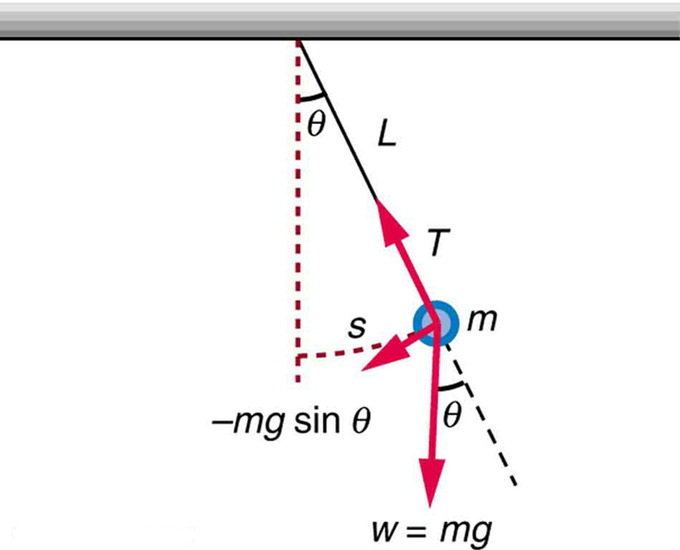Simple Pendulum: A simple pendulum has a small-diameter bob and a string that has a very small mass but is strong enough not to stretch appreciably. The linear displacement from equilibrium is s, the length of the arc. Also shown are the forces on the bob, which result in a net force of −mgsinθ toward the equilibrium position—that is, a restoring force.

For small displacements, a pendulum is a simple harmonic oscillator. A simple pendulum is defined to have an object that has a small mass, also known as the pendulum bob, which is suspended from a wire or string of negligible mass, such as shown in the illustrating figure. Exploring the simple pendulum a bit further, we can discover the conditions under which it performs simple harmonic motion, and we can derive an interesting expression for its period.

Pendulums: A brief introduction to pendulums (both ideal and physical) for calculus-based physics students from the standpoint of simple harmonic motion.

We begin by defining the displacement to be the arc length s. We see from the figure that the net force on the bob is tangent to the arc and equals −mgsinθ. (The weight mg has components mgcosθ along the string and mgsinθ tangent to the arc. ) Tension in the string exactly cancels the component mgcosθ parallel to the string. This leaves a net restoring force drawing the pendulum back toward the equilibrium position at θ = 0.

Now, if we can show that the restoring force is directly proportional to the displacement, then we have a simple harmonic oscillator. In trying to determine if we have a simple harmonic oscillator, we should note that for small angles (less than about 15º), sinθθ (sinθ and θ differ by about 1% or less at smaller angles). Thus, for angles less than about 15º, the restoring force F is

$\text{F}\approx -\text{mg}\theta$

.

The displacement s is directly proportional to θ. When θ is expressed in radians, the arc length in a circle is related to its radius (L in this instance) by:

$\text{s}=\text{L}\theta$

so that

$\theta =\frac{\text{s}}{\text{L}}$

.

For small angles, then, the expression for the restoring force is:

$\text{F}\approx \frac{\text{mgL}}{\text{s}}$

.

This expression is of the form of Hooke’s Law:

$\text{F}\approx -\text{kx}$

where the force constant is given by k=mg/L and the displacement is given by x=s. For angles less than about 15º, the restoring force is directly proportional to the displacement, and the simple pendulum is a simple harmonic oscillator.

Using this equation, we can find the period of a pendulum for amplitudes less than about 15º. For the simple pendulum:

$\text{T}=2\pi \sqrt{\frac{\text{m}}{\text{k}}}=2\pi \sqrt{\frac{\text{m}}{\frac{\text{mg}}{\text{L}}}}$

.

Thus,

$\text{T}=2\pi \sqrt{\frac{\text{L}}{\text{g}}}$

or the period of a simple pendulum. This result is interesting because of its simplicity. The only things that affect the period of a simple pendulum are its length and the acceleration due to gravity. The period is completely independent of other factors, such as mass. Even simple pendulum clocks can be finely adjusted and accurate. Note the dependence of T on g. If the length of a pendulum is precisely known, it can actually be used to measure the acceleration due to gravity. If θ is less than about 15º, the period T for a pendulum is nearly independent of amplitude, as with simple harmonic oscillators. In this case, the motion of a pendulum as a function of time can be modeled as:

$\theta \left(\text{t}\right)={\theta }_{\text{o}}\text{cos}\left(\frac{2\pi \text{t}}{\text{T}}\right)$

For amplitudes larger than 15º, the period increases gradually with amplitude so it is longer than given by the simple equation for T above. For example, at an amplitude of θ0 = 23° it is 1% larger. The period increases asymptotically (to infinity) as θ0 approaches 180°, because the value θ0 = 180° is an unstable equilibrium point for the pendulum.

## The Physical Pendulum

The period of a physical pendulum depends upon its moment of inertia about its pivot point and the distance from its center of mass.

### LEARNING OBJECTIVES

Identify parameters that affect the period of a physical pendulum

### KEY TAKEAWAYS

#### Key Points

• A physical pendulum is the generalized case of the simple pendulum. It consists of any rigid body that oscillates about a pivot point.
• For small amplitudes, the period of a physical pendulum only depends on the moment of inertia of the body around the pivot point and the distance from the pivot to the body’s center of mass. It is calculated as:
$\text{T}=2\pi \sqrt{\frac{\text{I}}{\text{mgh}}}$

.

• The period is still independent of the total mass of the rigid body. However, it is not independent of the mass distribution of the rigid body. A change in shape, size, or mass distribution will change the moment of inertia and thus, the period.

#### Key Terms

• physical pendulum: A pendulum where the rod or string is not massless, and may have extended size; that is, an arbitrarily-shaped, rigid body swinging by a pivot. In this case, the pendulum’s period depends on its moment of inertia around the pivot point.
• mass distribution: Describes the spatial distribution, and defines the center, of mass in an object.

### The Physical Pendulum

Recall that a simple pendulum consists of a mass suspended from a massless string or rod on a frictionless pivot. In that case, we are able to neglect any effect from the string or rod itself. In contrast, a physical pendulum (sometimes called a compound pendulum) may be suspended by a rod that is not massless or, more generally, may be an arbitrarily-shaped, rigid body swinging by a pivot (see ). In this case, the pendulum’s period depends on its moment of inertia around the pivot point.

Pendulums – Physical Pendulum: A brief introduction to pendulums (both ideal and physical) for calculus-based physics students from the standpoint of simple harmonic motion.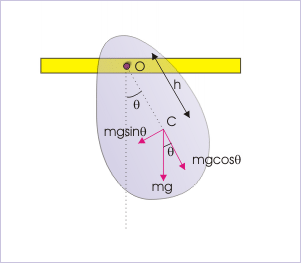A Physical Pendulum: An example showing how forces act through center of mass. We can calculate the period of this pendulum by determining the moment of inertia of the object around the pivot point.

Gravity acts through the center of mass of the rigid body. Hence, the length of the pendulum used in equations is equal to the linear distance between the pivot and the center of mass (h).

The equation of torque gives:

$\tau =\text{I}\alpha$

,

where α is the angular acceleration, τ is the torque, and I is the moment of inertia.

The torque is generated by gravity so:

$\tau =\text{mghsin}\theta$

,

where h is the distance from the center of mass to the pivot point and θ is the angle from the vertical.

Hence, under the small-angle approximation sin\theta \approx \theta,

$\alpha \approx -\frac{\text{mgh}\theta }{\text{I}}$

.

This is of the same form as the conventional simple pendulum and this gives a period of:

$\text{T}=2\pi \sqrt{\frac{\text{I}}{\text{mgh}}}$

.

And a frequency of:

$\text{f}=\frac{1}{\text{T}}=\frac{1}{2\pi }\sqrt{\frac{\text{mgh}}{\text{I}}}$

.

In case we know the moment of inertia of the rigid body, we can evaluate the above expression of the period for the physical pendulum. For illustration, let us consider a uniform rigid rod, pivoted from a frame as shown (see ). Clearly, the center of mass is at a distance L/2 from the point of suspension: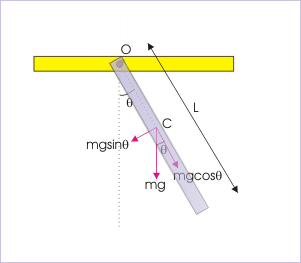Uniform Rigid Rod: A rigid rod with uniform mass distribution hangs from a pivot point. This is another example of a physical pendulum.

$\text{h}=\frac{\text{L}}{2}$

.

The moment of inertia of the rigid rod about its center is:

${\text{I}}_{\text{c}}=\frac{{\text{mL}}^{2}}{12}$

.

However, we need to evaluate the moment of inertia about the pivot point, not the center of mass, so we apply the parallel axis theorem:

${\text{I}}_{\text{o}}={\text{I}}_{\text{c}}+{\text{mh}}^{2}=\frac{{\text{mL}}^{2}}{12}+\text{m}\left(\frac{\text{L}}{2}{\right)}^{2}=\frac{{\text{mL}}^{2}}{3}$

.

Plugging this result into the equation for period, we have:

$\text{T}=2\pi \sqrt{\frac{\text{I}}{\text{mgh}}}=2\pi \sqrt{\frac{2{\text{mL}}^{2}}{3\text{mgL}}}=2\pi \sqrt{\frac{2\text{L}}{3\text{g}}}$

.

The important thing to note about this relation is that the period is still independent of the mass of the rigid body. However, it is not independent of the mass distribution of the rigid body. A change in shape, size, or mass distribution will change the moment of inertia. This, in turn, will change the period.

As with a simple pendulum, a physical pendulum can be used to measure g.

## Energy in a Simple Harmonic Oscillator

The total energy in a simple harmonic oscillator is the constant sum of the potential and kinetic energies.

### LEARNING OBJECTIVES

Explain why the total energy of the harmonic oscillator is constant

### KEY TAKEAWAYS

#### Key Points

• The sum of the kinetic and potential energies in a simple harmonic oscillator is a constant, i.e., KE+PE=constant. The energy oscillates back and forth between kinetic and potential, going completely from one to the other as the system oscillates.
• In a spring system, the conservation equation is written as:
$\frac{1}{2}{\text{mv}}^{2}+\frac{1}{2}{\text{kx}}^{2}=constant=\frac{1}{2}{\text{kX}}^{2}$

, where X is the maximum displacement.

• The maximum velocity depends on three factors: amplitude, the stiffness factor, and mass:
${\text{v}}_{\text{max}}=\sqrt{\frac{\text{k}}{\text{m}}}\text{X}$

.

#### Key Terms

• elastic potential energy: The energy stored in a deformable object, such as a spring.
• dissipative forces: Forces that cause energy to be lost in a system undergoing motion.

### Energy in a Simple Harmonic Oscillator

To study the energy of a simple harmonic oscillator, we first consider all the forms of energy it can have. Recall that the potential energy (PE), stored in a spring that follows Hooke’s Law is:

$\text{PE}=\frac{1}{2}{\text{kx}}^{2}$

,

where PE is the potential energy, k is the spring constant, and x is the magnitude of the displacement or deformation. Because a simple harmonic oscillator has no dissipative forces , the other important form of energy is kinetic energy (KE). Conservation of energy for these two forms is:

$\text{KE}+\text{PE}=\text{constant}$

,

which can be written as:

$\frac{1}{2}{\text{mv}}^{2}+\frac{1}{2}{\text{kx}}^{2}=\text{constant}$

.

This statement of conservation of energy is valid for all simple harmonic oscillators, including ones where the gravitational force plays a role. For example, for a simple pendulum we replace the velocity with v=, the spring constant with k=mg/L, and the displacement term with x=. Thus:

$\frac{1}{2}{\text{mL}}^{2}{\omega }^{2}+\frac{1}{2}\text{mgL}{\theta }^{2}=\text{constant}$

.

In the case of undamped, simple harmonic motion, the energy oscillates back and forth between kinetic and potential, going completely from one to the other as the system oscillates. So for the simple example of an object on a frictionless surface attached to a spring, as shown again (see ), the motion starts with all of the energy stored in the spring. As the object starts to move, the elastic potential energy is converted to kinetic energy, becoming entirely kinetic energy at the equilibrium position. It is then converted back into elastic potential energy by the spring, the velocity becomes zero when the kinetic energy is completely converted, and so on. This concept provides extra insight here and in later applications of simple harmonic motion, such as alternating current circuits.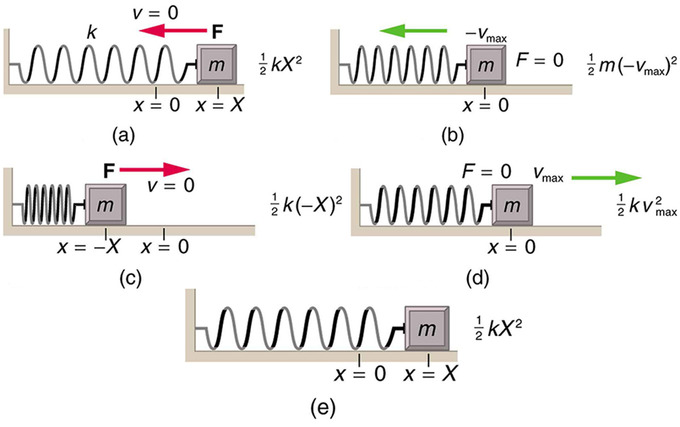Energy in a Simple Harmonic Oscillator: The transformation of energy in simple harmonic motion is illustrated for an object attached to a spring on a frictionless surface. (a) The mass has achieved maximum displacement from equilibrium. All energy is potential energy. (b) As the mass passes through the equilibrium point with maximum speed all energy in the system is in kinetic energy. (c) Once again, all energy is in the potential form, stored in the compression of the spring (in the first panel the energy was stored in the extension of the spring). (d) Passing through equilibrium again all energy is kinetic. (e) The mass has completed an entire cycle.

The conservation of energy principle can be used to derive an expression for velocity v. If we start our simple harmonic motion with zero velocity and maximum displacement (x=X), then the total energy is:

$\text{E}=\frac{1}{2}{\text{kX}}^{2}$

.

This total energy is constant and is shifted back and forth between kinetic energy and potential energy, at most times being shared by each. The conservation of energy for this system in equation form is thus:

$\frac{1}{2}{\text{mv}}^{2}+\frac{1}{2}{\text{kx}}^{2}=\frac{1}{2}{\text{kX}}^{2}$

.

Solving this equation for v yields:

$\text{v}=±\sqrt{\frac{\text{k}}{\text{m}}\left({\text{X}}^{2}-{\text{x}}^{2}\right)}$

.

Manipulating this expression algebraically gives:

$\text{v}=±\sqrt{\frac{\text{k}}{\text{m}}}\text{X}\sqrt{1-\frac{{\text{x}}^{2}}{{\text{X}}^{2}}}$

,

and so:

$\text{v}=±{\text{v}}_{\text{max}}\sqrt{1-\frac{{\text{x}}^{2}}{{\text{X}}^{2}}}$

,

where:

${\text{v}}_{\text{max}}=\sqrt{\frac{\text{k}}{\text{m}}}\text{X}$

.

From this expression, we see that the velocity is a maximum (vmax) at x=0. Notice that the maximum velocity depends on three factors. It is directly proportional to amplitude. As you might guess, the greater the maximum displacement, the greater the maximum velocity. It is also greater for stiffer systems because they exert greater force for the same displacement. This observation is seen in the expression for vmax; it is proportional to the square root of the force constant k. Finally, the maximum velocity is smaller for objects that have larger masses, because the maximum velocity is inversely proportional to the square root of m. For a given force, objects that have large masses accelerate more slowly.

A similar calculation for the simple pendulum produces a similar result, namely:

${\omega }_{\text{max}}=\sqrt{\frac{\text{g}}{\text{L}}}{\theta }_{\text{max}}$

.

Experience with a simple harmonic oscillator: A known mass is hung from a spring of known spring constant and allowed to oscillate. The time for one oscillation (period) is measured. This value is compared to a predicted value, based on the mass and spring constant.

## Sinusoidal Nature of Simple Harmonic Motion

The solutions to the equations of motion of simple harmonic oscillators are always sinusoidal, i.e., sines and cosines.

### LEARNING OBJECTIVES

Review factors responsible for the sinusoidal behavior of uniform circular motion

### KEY TAKEAWAYS

#### Key Points

• For simple harmonic oscillators, the equation of motion is always a second order differential equation that relates the acceleration and the displacement. The relevant variables are x, the displacement, and k, the spring constant.
• Solving the differential equation above always produces solutions that are sinusoidal in nature. For example, x(t), v(t), a(t), K(t), and U(t) all have sinusoidal solutions for simple harmonic motion.
• Uniform circular motion is also sinusoidal because the projection of this motion behaves like a simple harmonic oscillator.

#### Key Terms

• sinusoidal: In the form of a wave, especially one whose amplitude varies in proportion to the sine of some variable (such as time).

### Why are sine waves so common?

If the mass -on-a-spring system discussed in previous sections were to be constructed and its motion were measured accurately, its xt graph would be a near-perfect sine-wave shape, as shown in. It is called a “sine wave” or “sinusoidal” even if it is a cosine, or a sine or cosine shifted by some arbitrary horizontal amount. It may not be surprising that it is a wiggle of this general sort, but why is it a specific mathematically perfect shape? Why is it not a sawtooth shape, like in (2); or some other shape, like in (3)? It is notable that a vast number of apparently unrelated vibrating systems show the same mathematical feature. A tuning fork, a sapling pulled to one side and released, a car bouncing on its shock absorbers, all these systems will exhibit sine-wave motion under one condition: the amplitude of the motion must be small.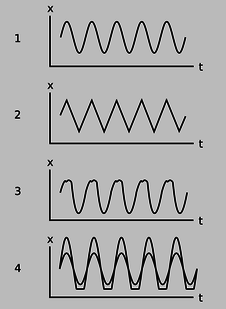Sinusoidal and Non-Sinusoidal Vibrations: Only the top graph is sinusoidal. The others vary with constant amplitude and period, but do no describe simple harmonic motion.

### Hooke’s Law and Sine Wave Generation

The key to understanding how an object vibrates is to know how the force on the object depends on the object’s position. If a system follows Hooke’s Law, the restoring force is proportional to the displacement. As touched on in previous sections, there exists a second order differential equation that relates acceleration and displacement.

${\text{F}}_{\text{net}}=\text{m}\frac{{\text{d}}^{2}\text{x}}{{\text{dt}}^{2}}=-\text{kx}$

.

When this general equation is solved for the position, velocity and acceleration as a function of time:

• $\text{x}\left(\text{t}\right)=\text{Acos}\left(\omega \text{t}-\phi \right)$

• $\text{v}\left(\text{t}\right)=\frac{\text{dx}}{\text{dt}}=-\text{A}\omega \text{sin}\left(\omega \text{t}-\phi \right)$

• $\text{a}\left(\text{t}\right)=\frac{{\text{d}}^{2}\text{x}}{{\text{dt}}^{2}}=-\text{A}{\omega }^{2}\text{cos}\left(\omega \text{t}-\phi \right)$

These are all sinusoidal solutions. Consider a mass on a spring that has a small pen inside running across a moving strip of paper as it bounces, recording its movements.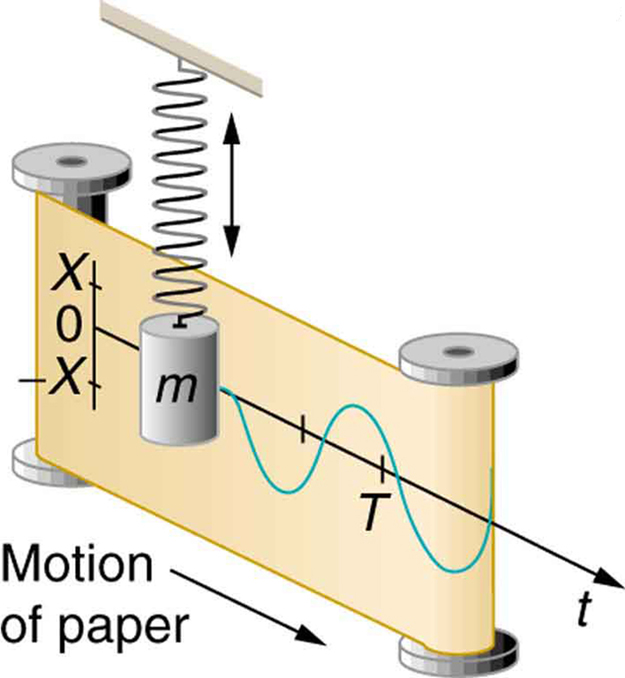Mass on Spring Producing Sine Wave: The vertical position of an object bouncing on a spring is recorded on a strip of moving paper, leaving a sine wave.

The above equations can be rewritten in a form applicable to the variables for the mass on spring system in the figure.

• $\text{x}\left(\text{t}\right)=\text{Xcos}\left(\frac{2\pi \text{t}}{\text{T}}\right)$

• $\text{v}\left(\text{t}\right)=-{\text{v}}_{\text{max}}\text{sin}\left(\frac{2\pi \text{t}}{\text{T}}\right)$

• $\text{a}\left(\text{t}\right)=-\frac{\text{kX}}{\text{m}}\text{cos}\left(\frac{2\pi \text{t}}{\text{T}}\right)$

Recall that the projection of uniform circular motion can be described in terms of a simple harmonic oscillator. Uniform circular motion is therefore also sinusoidal, as you can see from.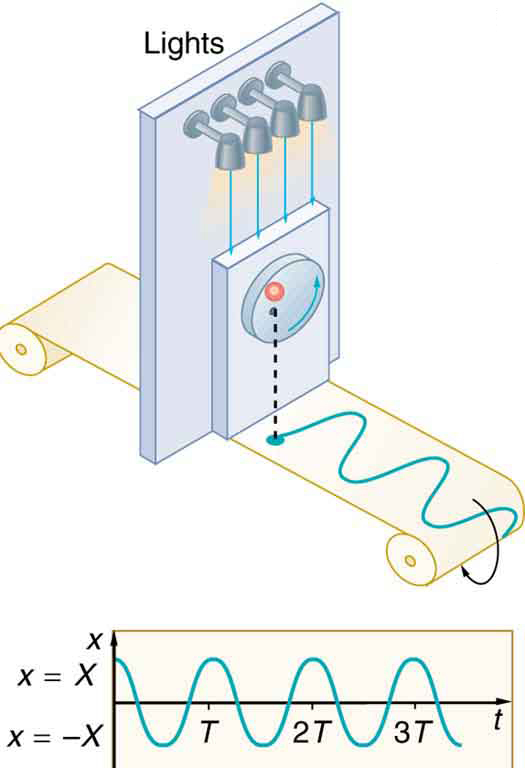Sinusoidal Nature of Uniform Circular Motion: The position of the projection of uniform circular motion performs simple harmonic motion, as this wavelike graph of x versus t indicates.

### Instantaneous Energy of Simple Harmonic Motion

The equations discussed for the components of the total energy of simple harmonic oscillators may be combined with the sinusoidal solutions for x(t)v(t), and a(t) to model the changes in kinetic and potential energy in simple harmonic motion.

The kinetic energy K of the system at time t is:

$\text{K}\left(\text{t}\right)=\frac{1}{2}{\text{mv}}^{2}\left(\text{t}\right)=\frac{1}{2}\text{m}{\omega }^{2}{\text{A}}^{2}{\text{sin}}^{2}\left(\omega \text{t}-\phi \right)=\frac{1}{2}{\text{kA}}^{2}{\text{sin}}^{2}\left(\omega \text{t}-\phi \right)$

.

The potential energy U is:

$\text{U}\left(\text{t}\right)=\frac{1}{2}{\text{kx}}^{2}\left(\text{t}\right)==\frac{1}{2}{\text{kA}}^{2}{\text{cos}}^{2}\left(\omega \text{t}-\phi \right)$

.

Summing K(t) and U(t) produces the total mechanical energy seen before:

$\text{E}=\text{K}+\text{U}=\frac{1}{2}{\text{kA}}^{2}$

.

Billing Information